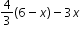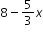# MA.7.AR.1.2

Determine whether two linear expressions are equivalent.

### Examples

Are the expressionsandequivalent?

### Clarifications

Clarification 1: Instruction includes using properties of operations accurately and efficiently.

Clarification 2: Instruction includes linear expressions in any form with rational coefficients.

Clarification 3: Refer to Properties of Operations, Equality and Inequality (Appendix D).

General Information
Subject Area: Mathematics (B.E.S.T.)
Strand: Algebraic Reasoning
Status: State Board Approved

## Related Courses

This benchmark is part of these courses.
1205020: M/J Accelerated Mathematics Grade 6 (Specifically in versions: 2014 - 2015, 2015 - 2020, 2020 - 2022, 2022 and beyond (current))
1205040: M/J Grade 7 Mathematics (Specifically in versions: 2014 - 2015, 2015 - 2022, 2022 and beyond (current))
1204000: M/J Foundational Skills in Mathematics 6-8 (Specifically in versions: 2014 - 2015, 2015 - 2022, 2022 and beyond (current))
7812020: Access M/J Grade 7 Mathematics (Specifically in versions: 2014 - 2015, 2015 - 2018, 2018 - 2019, 2019 - 2022, 2022 and beyond (current))

## Related Access Points

Alternate version of this benchmark for students with significant cognitive disabilities.
MA.7.AR.1.AP.2: Use tools or manipulatives to compare two linear expressions, with no more than two operations, to determine whether they are equivalent.

## Related Resources

Vetted resources educators can use to teach the concepts and skills in this benchmark.

## Formative Assessments

Rectangle Expressions:

Students are given equivalent expressions with rational coefficients and asked to explain what each expression represents within the context of the problem.

Type: Formative Assessment

Explain Equivalent Expressions:

Students are given equivalent expressions with rational coefficients and asked to explain what each expression represents within the context of a problem.

Type: Formative Assessment

Equivalent Rational Expressions:

Students are given a polynomial with rational coefficients and asked to identify equivalent expressions from a given list.

Type: Formative Assessment

Identify Equivalent Multistep Expressions:

Students are given an expression and are asked to identify expressions equivalent to it.

Type: Formative Assessment

## Original Student Tutorial

Math Soup: Creating Equivalent Expressions by Combining Like Terms :

Learn how to combine like terms to create equivalent expressions in this cooking-themed, interactive tutorial.

Type: Original Student Tutorial

## MFAS Formative Assessments

Equivalent Rational Expressions:

Students are given a polynomial with rational coefficients and asked to identify equivalent expressions from a given list.

Explain Equivalent Expressions:

Students are given equivalent expressions with rational coefficients and asked to explain what each expression represents within the context of a problem.

Identify Equivalent Multistep Expressions:

Students are given an expression and are asked to identify expressions equivalent to it.

Rectangle Expressions:

Students are given equivalent expressions with rational coefficients and asked to explain what each expression represents within the context of the problem.

## Original Student Tutorials Mathematics - Grades 6-8

Math Soup: Creating Equivalent Expressions by Combining Like Terms :

Learn how to combine like terms to create equivalent expressions in this cooking-themed, interactive tutorial.

## Student Resources

Vetted resources students can use to learn the concepts and skills in this benchmark.

## Original Student Tutorial

Math Soup: Creating Equivalent Expressions by Combining Like Terms :

Learn how to combine like terms to create equivalent expressions in this cooking-themed, interactive tutorial.

Type: Original Student Tutorial

## Parent Resources

Vetted resources caregivers can use to help students learn the concepts and skills in this benchmark.